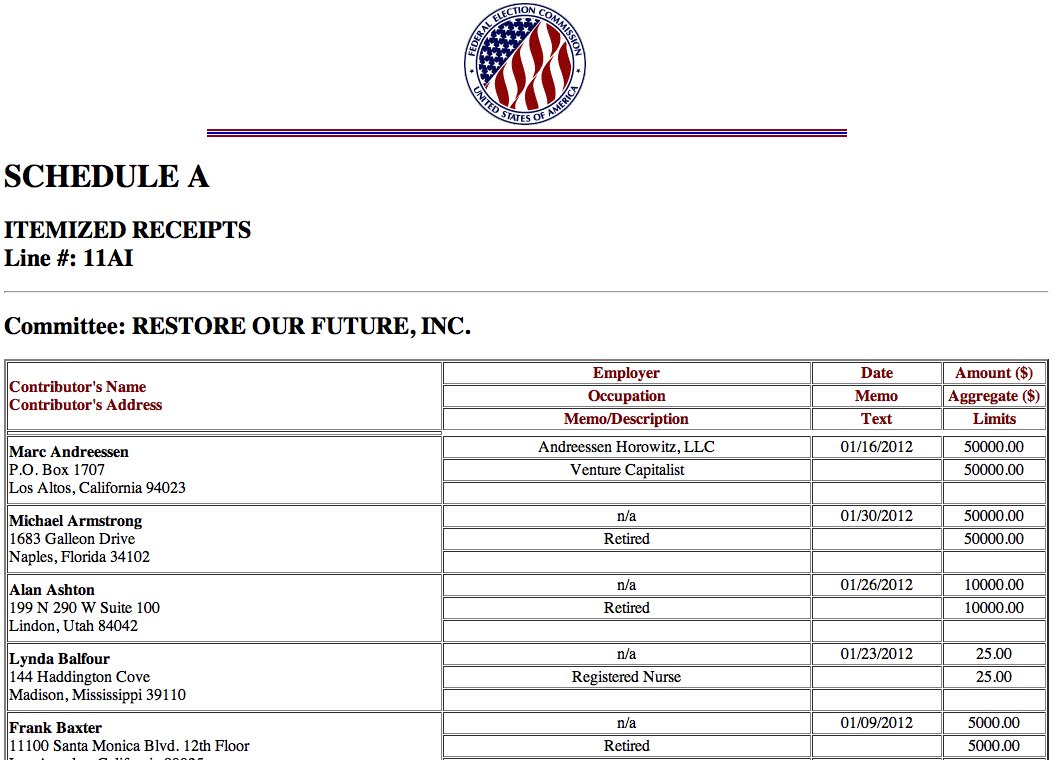## Ruby at NICAR15

← Use arrow keys →

## Coming up:2. Acquiring & Transforming Data with @thejefflarson3. Simple Web Apps with @harrisj

```Follow Along
http://j.mp/nicar-15-ruby
```

## Set up the room.

```ruby yourfile.rb
```
```\$ irb
irb(main):001:0> puts "hello"
hello
=> nil
```

## Assignment

```name = "Al"
```

## Conditional

```if name == "Al"
puts "Hello #{name}"
end
```

## Iteration

```names = ["Al", "Joe", "Sally"]
names.each do |name|
puts "#{name} is here!"
end
```

## Strings & Integers

```address  = 55
city     = "New York"
state    = "NY"
zip_code = "10006"
```
```city + ", " + state
#=> "New York, NY"
```
```"#{city}, #{state}"
#=> "New York, NY"
```
```"#{address} #{street}"
```
```address + " " + street
#=> TypeError: String can't be coerced into Fixnum
from (irb):10:in `+'
from (irb):10
```
```address.to_s + " " + street
```

## Type coercion

```5.to_s    #=> "5"
"5".to_i  #=> 5
5.to_f    #=> 5.0
```
```str = ""
str << city
str << ", "
str << state

#=> "New York, NY"
```
```str = ""
str += city
str += ", "
str += state

#=> "New York, NY"
```
```address += address
#=> 110
```
```= vs. ==
```
```a = 3
b = 3

a == b
#=> true
```
```a = 3
b = 3

if a == b
puts 'they match'
end
```
```a = 1
b = 2

if a != b
puts "They don't match!"
end
```
```a = 3
b = 3

if a == b
puts "they match"
else
puts "they don't match"
end
```

## Arrays & Hashes

```[]
```
```students = ["Amy", "Joe", "Sally", "Harry"]
```
```students.pop
#=> "Harry"

students
#=> ["Amy", "Joe", "Sally"]
```
```students.shift
#=> "Amy"

students
#=> ["Joe", "Sally"]
```
```students << "Amy"
#=> ["Joe", "Sally", "Amy"]
```
```students
#=> "Joe"
```
```students
#=> ?
```
```students
#=> "Sally"
```
```person = {}
```
```person = {}
person[:first_name] = "Al"
person[:last_name]  = "Shaw"
```
```person = {
:first_name => "Al",
:last_name  => "Shaw"
}
```
```person[:first_name]
#=> "Al"
```
```people = []
people.push(person)
#=> [{:first_name=>"Al", :last_name=>"Shaw"}]
```

## Iteration

```students.each do |student|
puts "#{student} is in class today"
end

#=>
Joe is in class today
Sally is in class today
Amy is in class today
```
```even_numbers = [2, 4, 6, 8, 10]
odd_numbers  = []
# how can we programmatically
# fill up this array with odd numbers
```
```even_numbers.each do |n|
odd_numbers << n + 1
end

odd_numbers
#=> [3, 5, 7, 9, 11]
```
```even_numbers.map do |n|
n + 1
end
#=> [3, 5, 7, 9, 11]
```
```even_numbers.map { |n| n + 1 }
#=> [3, 5, 7, 9, 11]
```
```even_numbers
#=> [2, 4, 6, 8, 10]

even_numbers.map! { |n| n + 1 }
#=> [3, 5, 7, 9, 11]
```

## Methods

```def add(a, b)
a + b
end
```
```add(1, 2)
#=> 3
```
```# Who's in class today?
def is_in_class(student)
attendance = {
"Amy"   => true,
"Joe"   => true,
"Sally" => false,
"Harry" => true
}

if attendance[student]
"here"
else
"absent"
end
end
```
```# Try it
is_in_class("Sally")
#=> Absent
is_in_class("Joe")
#=> Here
```

## Classes are factories for objects

```class Student
@name  = name
end
end
```
```s = Student.new("Sally", 8)
#=> #<Student:0x1052aca28 @name="Sally", @grade=8>

s.name
#=> "Sally"
```

## Let's build a system that assigns students to teachers!

```class Student
@name  = name
end

@@teachers = {
"Shanahan"  => 5,
"Kovacek"   => 5,
"Streich"   => 5,
"Ankunding" => 5,
"Marks"     => 5
}

def assign_teacher
@@teachers.each do |t_name, slots|
if slots > 0
@teacher = t_name
@@teachers[t_name] -= 1
puts "#{@name} is assigned to #{t_name}"
puts "#{t_name} now has #{slots - 1} slots left"
return
end
end
end
end
```
```s = Student.new("Sally", 8)
#=> #<Student:0x1052aca28 @name="Sally", @grade=8>

s.assign_teacher
Sally is assigned to Streich
Streich now has 4 slots left

s.teacher
#=> "Streich"
```

## Everything in Ruby is an object

```1.+(2)
#=> 3
```
```class Fixnum
def +(num)
self - num
end
end
```
```1 + 2
#=> -1
```

## A useful example:

```[1,2,3].sum
NoMethodError: undefined method `sum' for [1, 2, 3]:Array
from (irb):1
```
```class Array
def sum
self.reduce(0) {|memo, it| memo += it; memo}
end
end
```
```[1,2,3].sum
#=> 6
```

## RubyGems are bits of code that add functionality to Ruby

```// In Ruby 2.0, json is installed by default.
// If you don't have it, run
\$ gem install json
```
```require 'json'
```

## Let's use the JSON gem to play with the Facebook API

```require 'json'
require 'open-uri'
```
```JSON.parse(open("http://graph.facebook.com/ashaw").read)

{"gender"=>"male",
"id"=>"2902652",
"locale"=>"en_US",
"first_name"=>"Al",
"last_name"=>"Shaw",
"name"=>"Al Shaw",
```

## Finally, let's use Nokogiri to do some simple scraping

```\$ gem install nokogiri
```
```require 'nokogiri'
require 'open-uri'
```

## FEC.gov```url = "http://query.nictusa.com/cgi-bin/dcdev/forms/C00490045/766953/sa/11AI"

```
```names = []
donors = doc.css("table tr td[align=LEFT]")
donors.each do |donor|
name = donor.css("b").content
names << name
end
```
```names

#=>
["Marc Andreessen",
"Michael Armstrong",
"Alan Ashton",
"Lynda Balfour",
"Frank Baxter",
"Glen Beck",
"James Berardinelli",
...
]

```